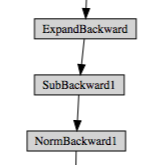# Incorrect computation graph?

Consider the following function with `p.requires_grad=True` (the other two parameters are constants):

``````   def projectionDown(self,p,cam,computeDepth=False):
if computeDepth:
depth = (p-torch.tensor(cam['centre'],dtype=torch.float,device=self.gpu)).norm(p=2,dim=0)
else:
depth = None
p = torch.cat((p,torch.ones(1,p.shape,device=self.gpu)),0)
res = torch.mm(torch.tensor(cam['P'],dtype=torch.float,device=self.gpu),p)
return res[:-1]/res[-1], depth
``````

The computation graph for this function looks like this:However, this only accounts for the line inside the if-statement. Clearly, the new `p` (in line 5 of the function body) and `res` also depend on the input parameter `p`, so the computation graph should branch. What’s going on?

You cropped the picture?
More seriously, it is very unlikely that PyTorch executed your calculation but forgot about it, maybe it is something about the tools you use or the broader context of your code, which you don’t say anything about.

Best regards

Thomas

I cropped the picture, because it is a big graph. I am 100% certain those lines are executed. The only reason I can think of is a bug in the visualization tool. Is there any way to check the computation graph manually during pdb.set_trace()?